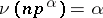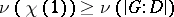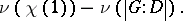Brauer height-zero conjecture

Letbe an irreducible character in a blockof a groupwith defect group(cf. also Defect group of a block). Letbe the discrete valuation defined on the integers withwheneveris prime to. By a theorem of Brauer,. The height ofis defined to beEvery block contains an irreducible character of height zero. Brauer's height-zero conjecture is the assertion that every irreducible character inhas height zero if and only ifis Abelian (cf. also Abelian group).
That every irreducible character inhas height zero whenis Abelian was proved for-solvable groups (cf. also-solvable group) by P. Fong (see [a2], X.4). The converse for-solvable groups was proved by D. Gluck and T. Wolf [a3], using the classification of finite simple groups. The "if" direction has been reduced to the consideration of quasi-simple groups by T.R. Berger and R. Knörr [a1]. The task of checking this half of the conjecture for the quasisimple groups was completed in 2011 by R. Kessar and G. Malle [a4], hence completing the proof of the "if" direction. The evidence for the "only if" direction is more slender.